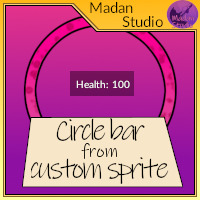# Circle bar from custom sprite

#### Morena Games

You must be logged in to obtain assets

### Description

Useful easy to use sprite health bar!

Use your custom sprites. All size supported.

Only one line of code!

How to use:

For increasing bars, like health:

``````draw_circlebar_increase(_sprite_index, _x, _y, _max_Value, _actual_Value, _draw_Background);
``````

Where:

_sprite_index - Is sprite_index of circle bar we want to use.

_x - X position where we want to draw bar

_y - Y position where we want to draw bar

_max_Value - Max value

_actual_Value - Actual value

_draw_Background - Whether you want to draw the background

For decreasing bars, like count down:

``````draw_circlebar_decrease(_sprite_index, _x, _y, _max_Value, _actual_Value, _draw_Background);
``````

_sprite_index - Is sprite_index of circle bar we want to use.

_x - X position where we want to draw bar

_y - Y position where we want to draw bar

_max_Value - Max value of time (60/one minute)

_actual_Value - Actual time value

_draw_Background - Whether you want to draw the background

All images origin needs to be centered!